# 利用51单片机制作一个秒表的详细过程

1 篇文章 0 订阅

## 1.整体思路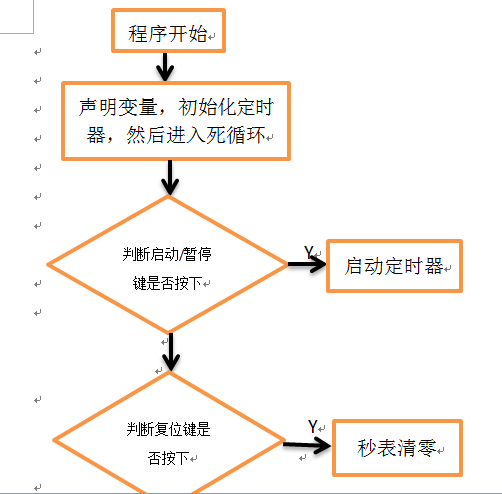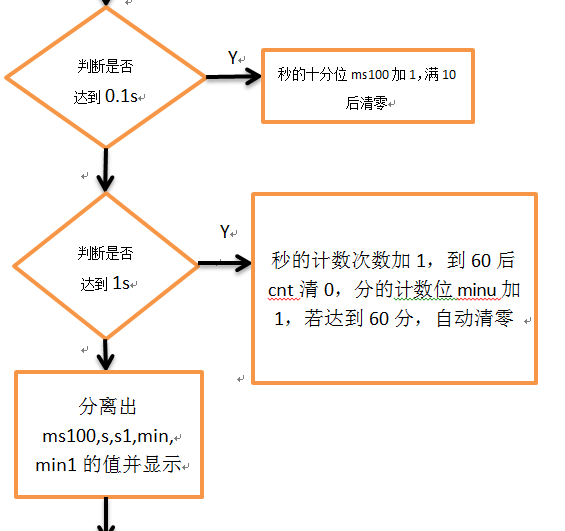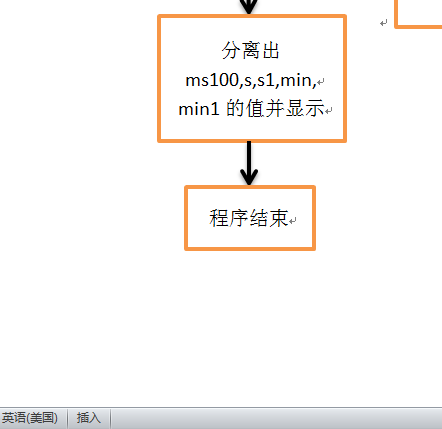“ms100”表示秒的十分位，"s"表示秒的个位，“s1”表示秒的十位，“min”表示分的个位，“min1”表示分的十位。
“cnt”表示秒的计数位，即多少个一秒，定时满一秒加1，“minu”表示分的计数位，即多少个一分钟，一分钟加1，

## 2.硬件电路方面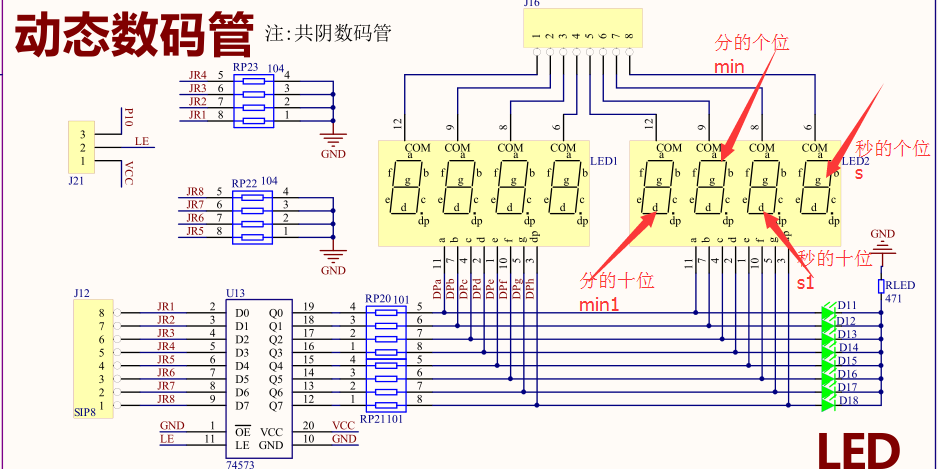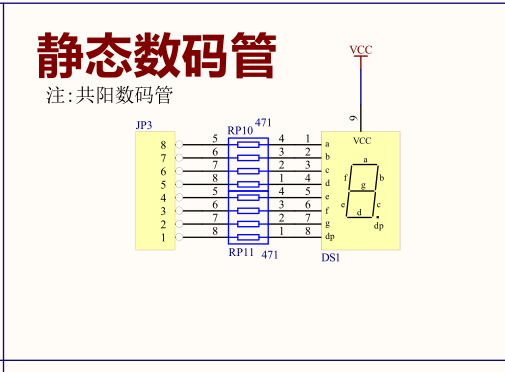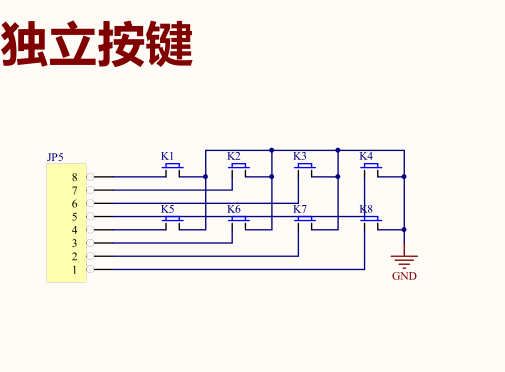## 3.软件程序方面

#include <REGX51.H>
#define uchar unsigned char
#define uint unsigned int

#define PIN0 P0  //数码管段选端
#define PIN1 P1  //数码管位选端
#define PIN3 P3	 //十分位数码管的段码端

sbit start=P2^7;  //启动/暂停按钮
sbit reset=P2^6;  //复位按钮

uint cnt=0;   //1s的次数的计数值
uint num=0;     //进入中断次数的计数值（一次50ms）
uint num1=0;		//0.1s的次数的计数值

uchar code table[]={0x3f,0x06,0x5b,
0x4f,0x66,0x6d,0x7d,0x07, 0x7f,0x6f};
//不带小数点的共阴极数码管段码0-9


uchar code table1[]={0xbf,0x86,
0xdb,0xcf,0xe6,0xed,0xfd,0x87,0xff,0xef};
//带小数点的共阴极数码管段码0-9

char code table2[]={0xc0,0xf9,0xa4,
0xb0,0x99,0x92,0x82,0xf8,0x80,0x90};
//不带小数点的共阳极数码管段码0-9

void Display(uint w,uint a,uint b,uint c,uint d);  //声明显示函数
void InitTimer0();  //声明定时器初始化函数
void Delayms(uint x);  //声明延时函数

void main()
{
uint ms100=0;	//秒的十分位
uint s,s1,min,min1,minu=0;
//依次是秒的个位，秒的十位，分的个位，分的十位
InitTimer0();	  //初始化定时器
while(1)			//进入死循环
{
if(start==0)   //检测启动/暂停键是否按下
Delayms(20); //延时消抖
{
if(start==0)  //消抖之后再次检测
{
TR0=！TR0;		//定时器的控制位取反
while(!start);  //等待按键释放
}
}

if(reset==0)  //检测复位键是否按下
{
Delayms(20);	//延时消抖
if(reset==0)  	//消抖之后再次检测
{
num=0;
num1=0;		//进入中断次数值清0
ms100=0;	//十分位的计数值清0
cnt=0;		//秒的计数值清0
minu=0;		//分的计数值清0
while(!reset);		 //等待按键释放
}
}
if(num1>=2)		//检测是否达到0.1s,（两个50ms）
{
num1=0;  		//进入中断次数值清零
ms100++;		//秒的十分位的计数值加1
if(ms100>=10)  //若秒的十分位计数值达到10
{
ms100=0;  //清零秒的十分位的计数值
num1=0;	//进入中断次数值置0
}
}

if(num>=20)  //判断计时时间是否达到1s（20个50ms）
{
num=0;     //进入中断次数值置0
cnt++;		 //秒的计数值加1
if(cnt>=60) //判断是否达到60s
{
cnt=0;		//若达到60s，将秒的计数值置零
minu++;			//分的计数值加1
if(minu>=60)  //判断是否达到60分钟，一般秒表是用不到60分的，这是为了程序的严谨性

{
TR0=!TR0;			//达到60分钟则关闭定时器并清零所有的计数值
num1=0;
num=0;
ms100=0;
cnt=0;
minu=0;

}
}
}
s=cnt%10;  	//从秒的计数值里面分离秒的个位
s1=cnt/10; 	//从秒的计数值里面分离秒的十位
min=minu%10;	//从分的计数值里面分离分的个位
min1=minu/10;	//从分的计数值里面分离分的十位

Display(ms100,s,s1,min,min1);  //显示这5个数
}
}

void InitTimer0() //定时器初始化函数
{

TMOD=0x01; //选择定时器0的工作方式1
TH0=(65536-45872)/256;  //装初值（定时50ms）,晶振11.0592MHz
TL0=(65536-45872)%256;
EA=1;		//打开总中断
ET0=1;	//打开定时器中断
TR0=0;	//先不要启动定时器

}

void TIMER0()  interrupt 1  //定时器中断服务函数
{
TH0=(65536-45872)/256;  //重装初值
TL0=(65536-45872)%256;
num++;		//让进入中断次数值加1，用于判断是否达到1s
num1++;		//让进入中断次数值加1，用于判断是否达到0.1s
}

void Delayms(uint x)   //延时xms
{
uint i,j;
for(i=x;i>0;i--)
for(j=110;j>0;j--);
}

void Display(uint w,uint a,uint b,uint c,uint d) //定义显示函数
{
PIN3=table2[w];		//秒的十分位的段选
Delayms(5);

PIN0=table[a]; //秒的个位的段选
PIN1=0x7f;		 //秒的个位的位选
Delayms(5);

PIN0=table[b];  //秒的十位的段选
PIN1=0xbf;			//秒的十位的位选
Delayms(5);

PIN0=table1[c];  //分的个位的段选（带小数点）
PIN1=0xdf;			 //分的个位的位选
Delayms(5);

PIN0=table[d];		//分的十位的段选
PIN1=0xef;				//分的十位的位选
Delayms(5);

}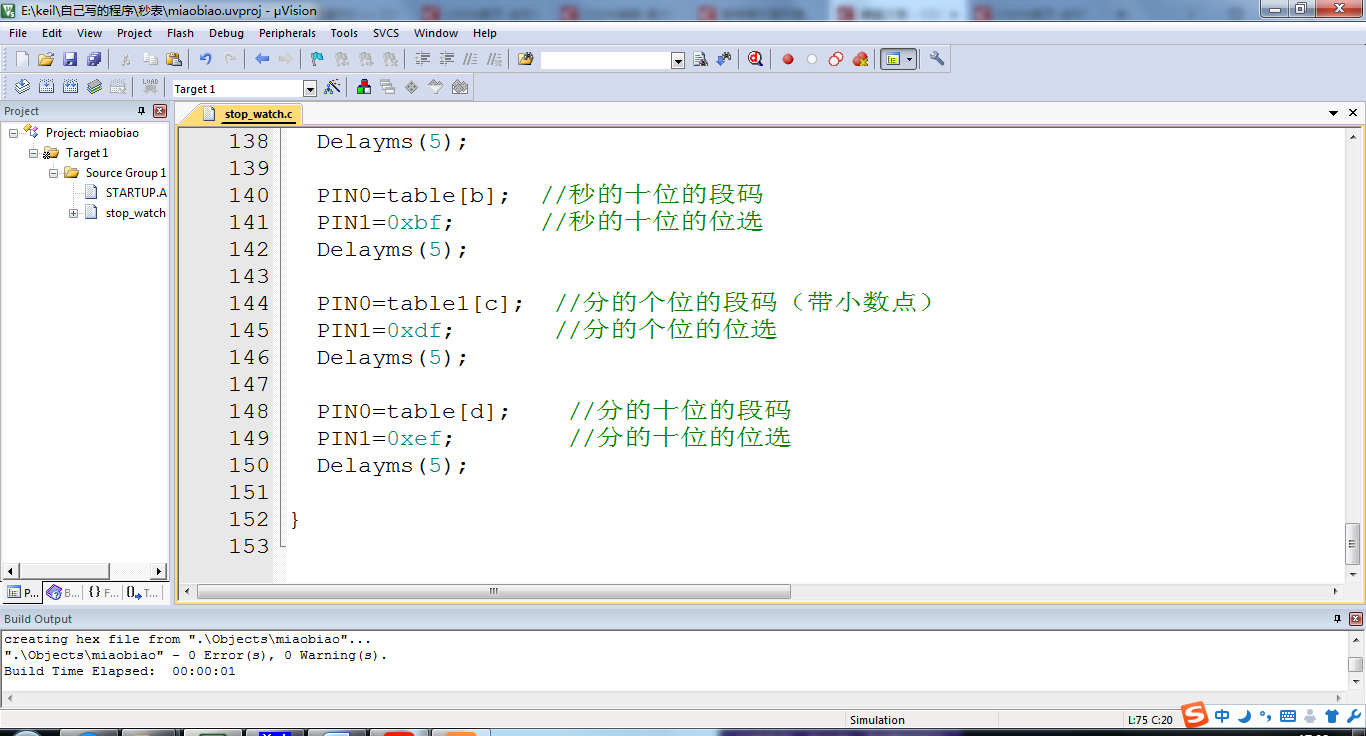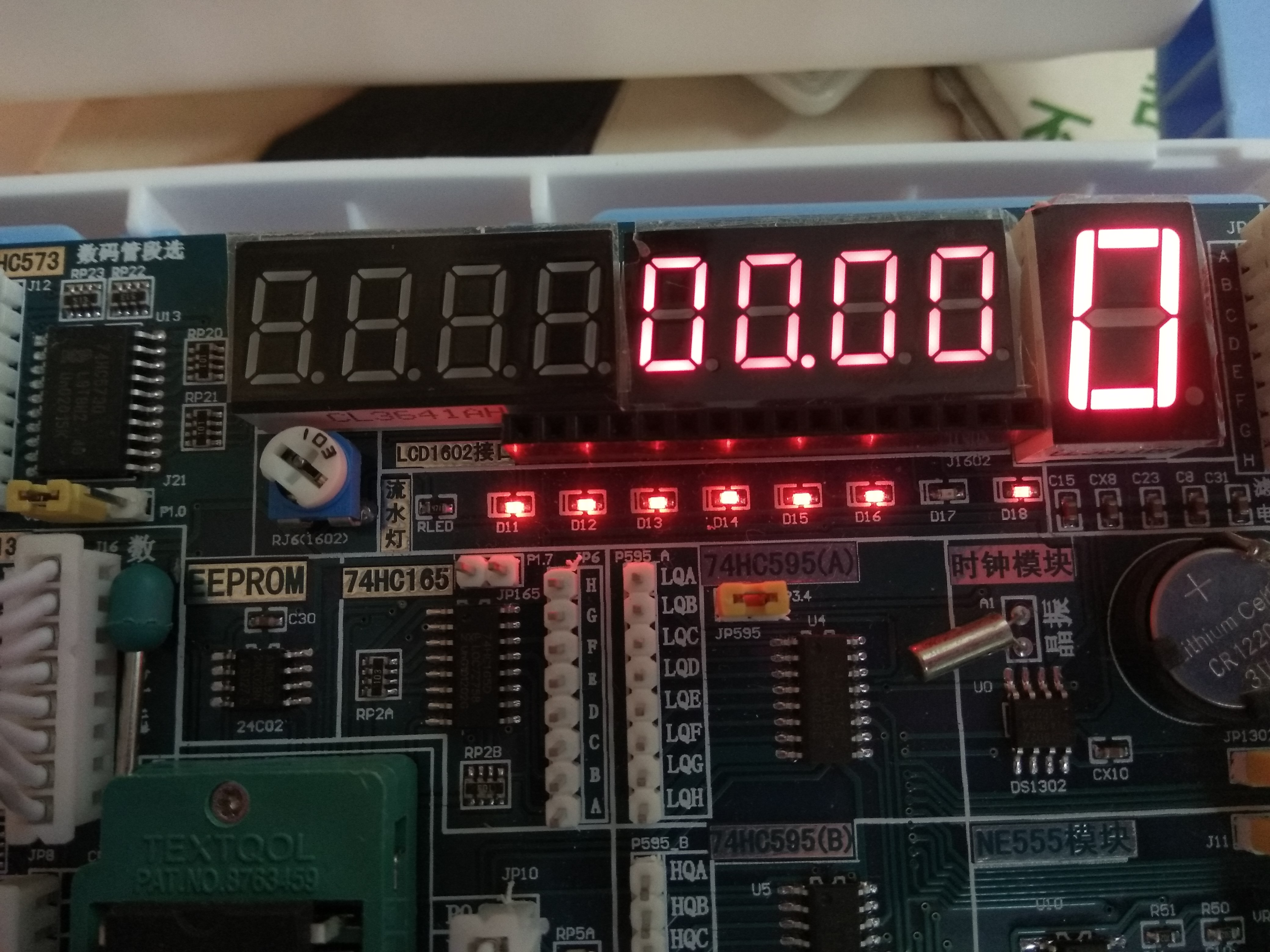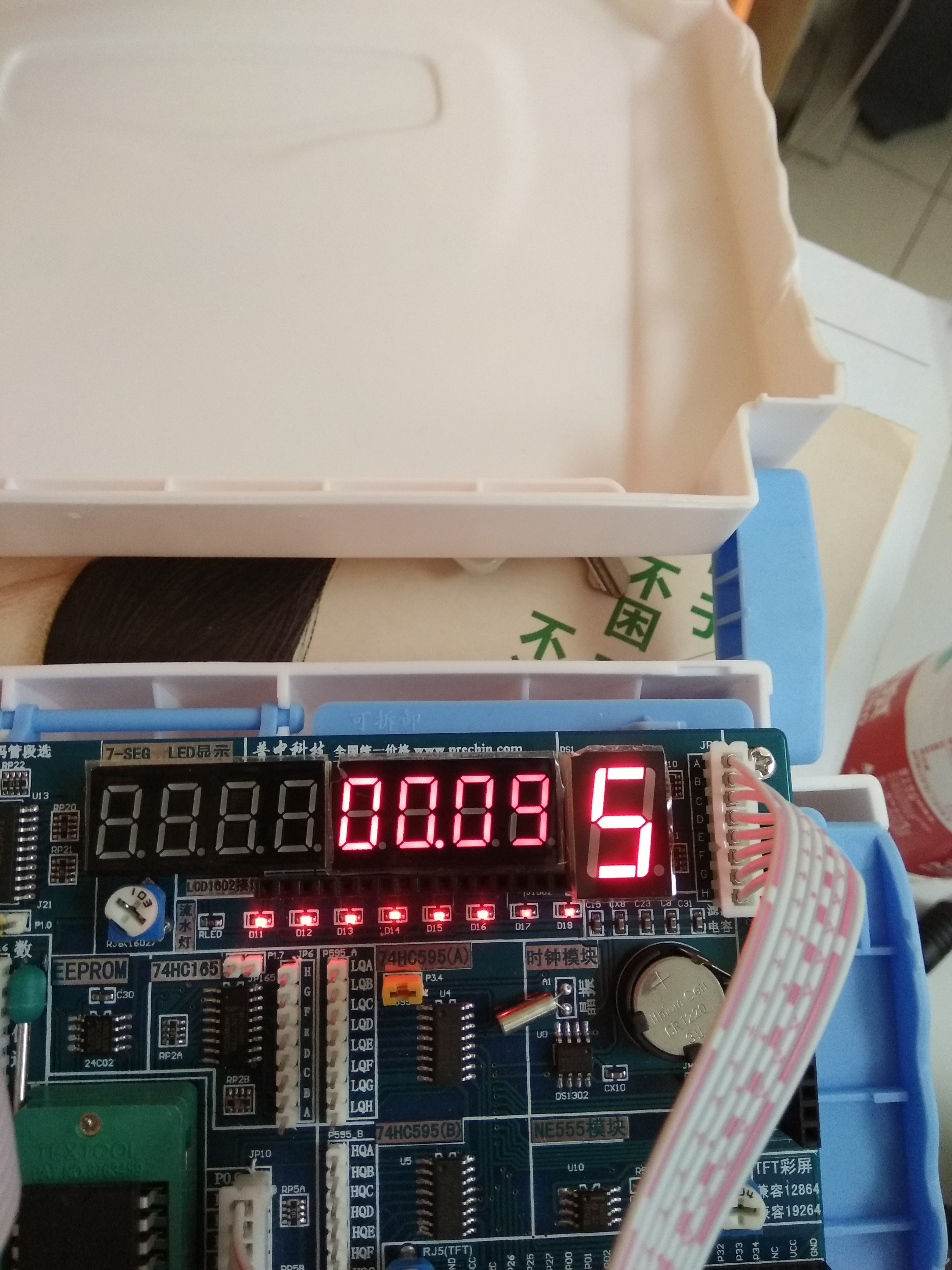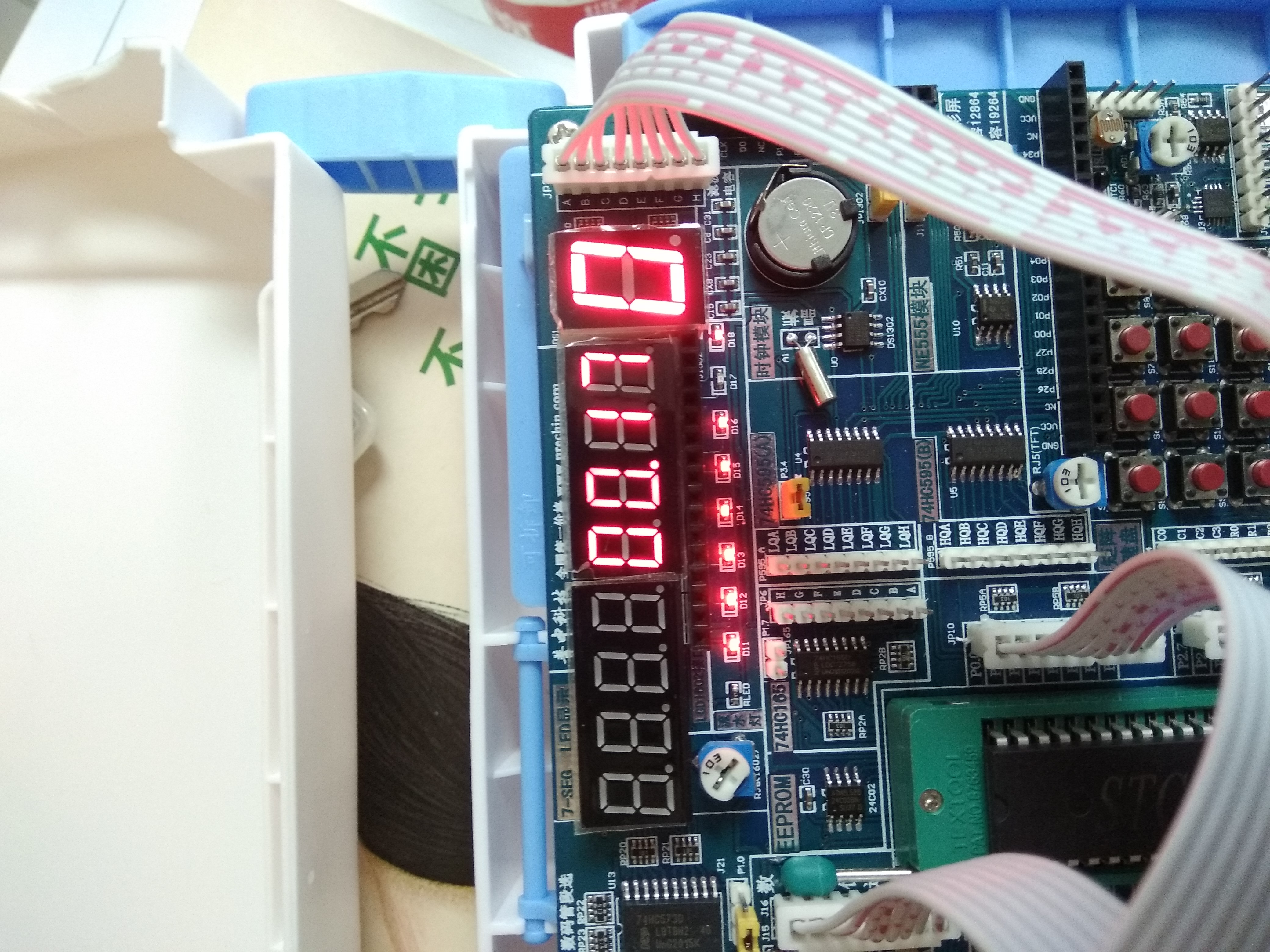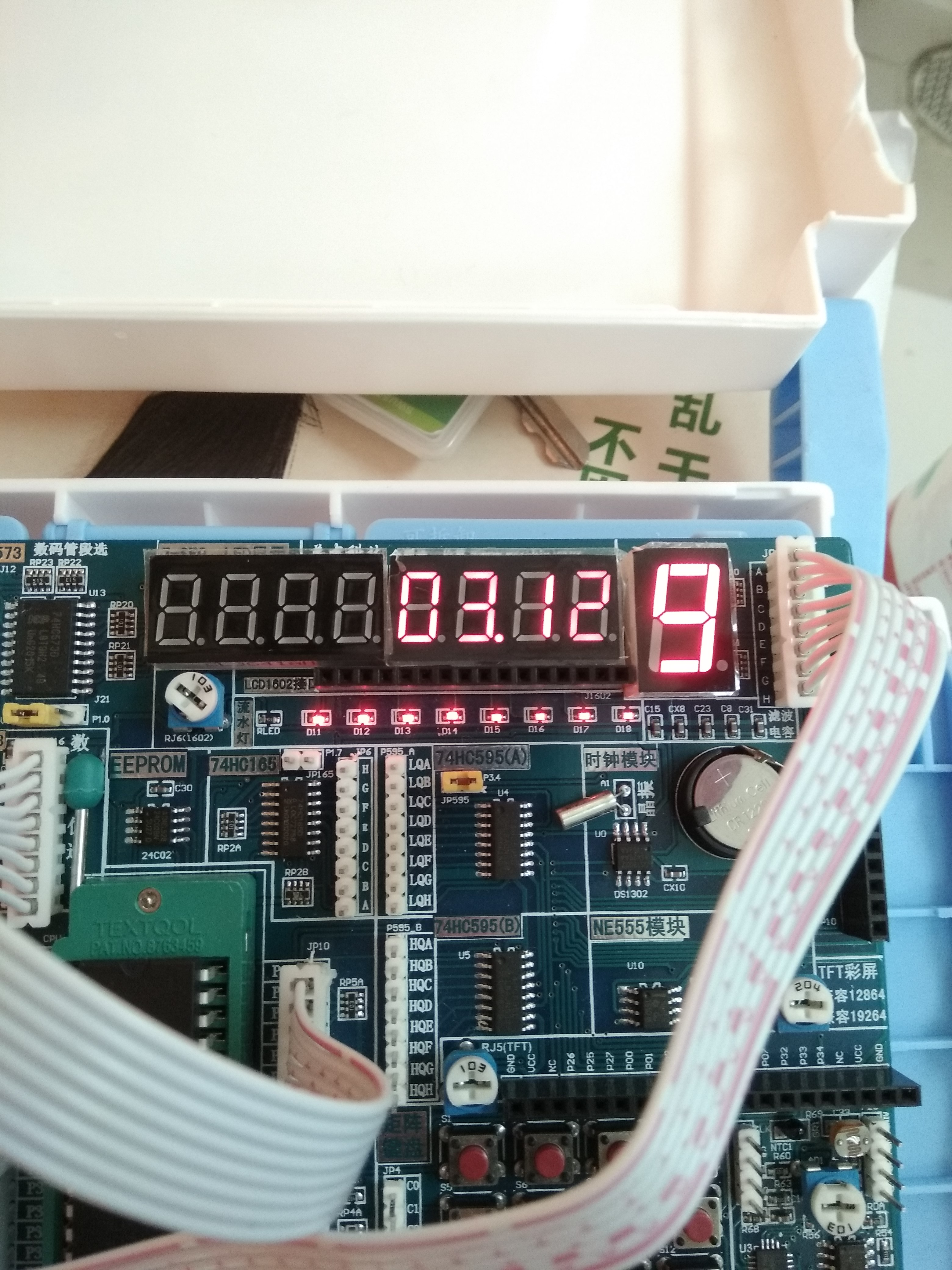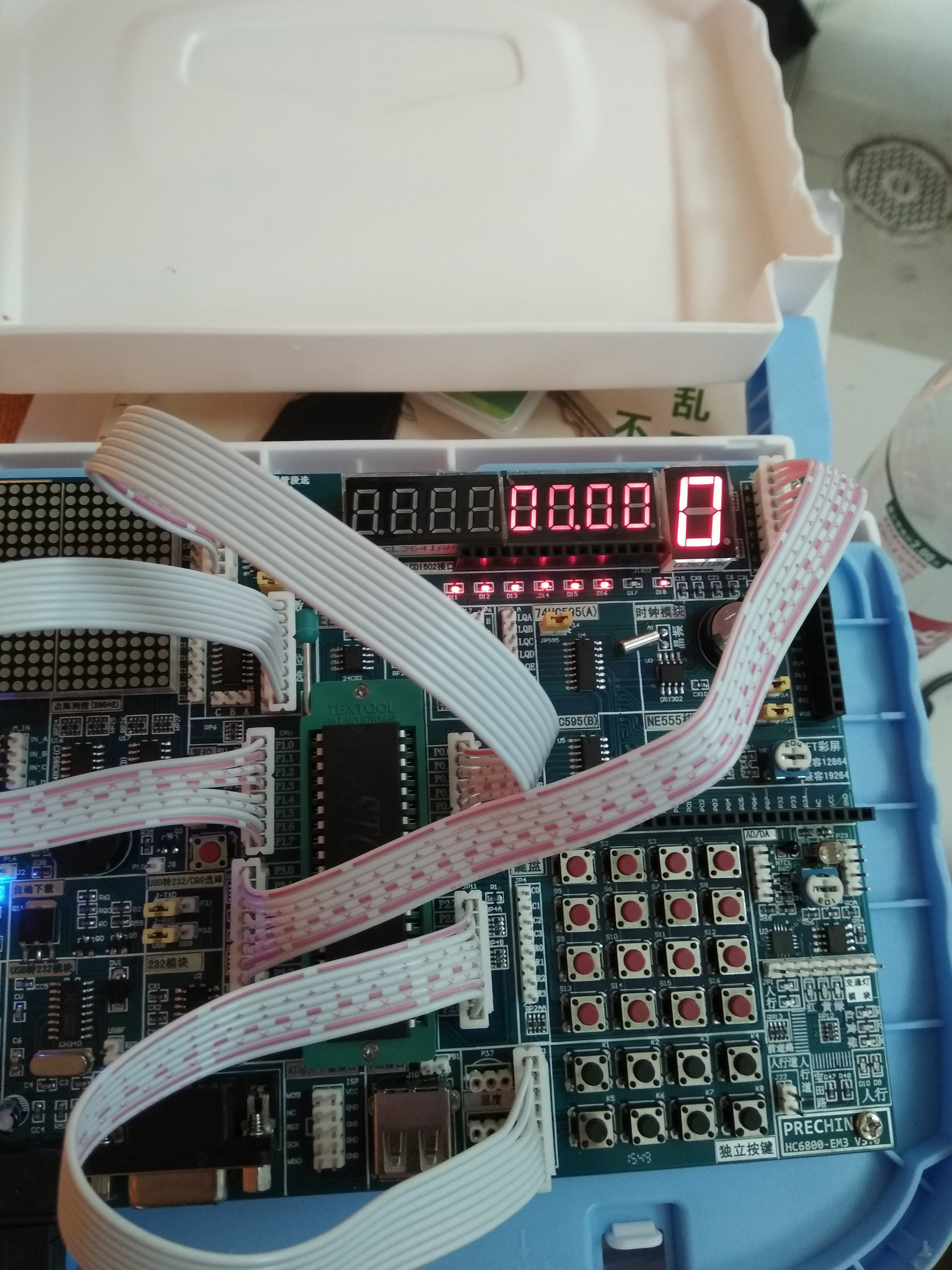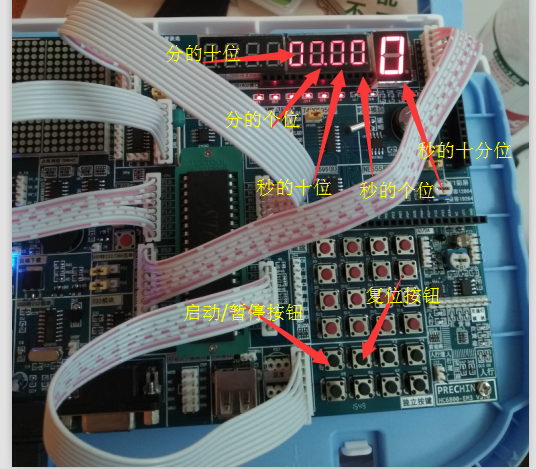## 结束语

（PS：我写了一整天了，反复的看，反复的改，现在头昏眼花，但是只要能对大家有所帮助，一切都是值得的。）

05-2905-06
05-18182
01-111万+
06-061256
11-279726
09-081万+
04-254867
01-251万+
05-08
11-19
05-21
11-293110
05-189584
04-20606点击重新获取扫码支付余额充值